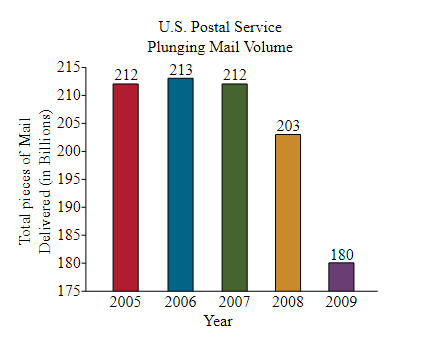Chapter 1.II, Problem 28RE### Contemporary Mathematics for Busin...

8th Edition
Robert Brechner + 1 other
ISBN: 9781305585447

#### Solutions

Chapter
Section### Contemporary Mathematics for Busin...

8th Edition
Robert Brechner + 1 other
ISBN: 9781305585447
Textbook Problem

# Use the U.S Postal Service Mail Volume graph on the next page to answer the following questions.a. How many pieces were delivered in 2005 and 2006 combined?b. How many fewer pieces were delivered in 2009 than in 2007?c. Write the number of pieces of mail from 2008 in numerical form.

(a)

To determine

To calculate: The pieces of postal mail delivered in 2005 and 2006 combined by using the following graph:Explanation

Given information:

The given mail volume graph is,

Formula used:

Use the following steps to add the numbers.

Step 01: First write all the numbers in columns such that all the place values are aligned.

Step 02: Add the digits in each column, starting from the right.

If the total is a two-digit number then write its unit digit under the column which is added and carry the tens digit to the column to the left.

Calculation:

Consider the provided graph:

To find the numbers of postal mail delivered in 2005 and 2006 combined, check the number written over the bar of year 2005 and 2006

(b)

To determine

To calculate: The number of fewer pieces which were delivered in 2009 than in 2007.

(c)

To determine

The numerical form of the number of the mails delivered in 2008.

### Still sussing out bartleby?

Check out a sample textbook solution.

See a sample solution

#### The Solution to Your Study Problems

Bartleby provides explanations to thousands of textbook problems written by our experts, many with advanced degrees!

Get Started

#### Find more solutions based on key concepts